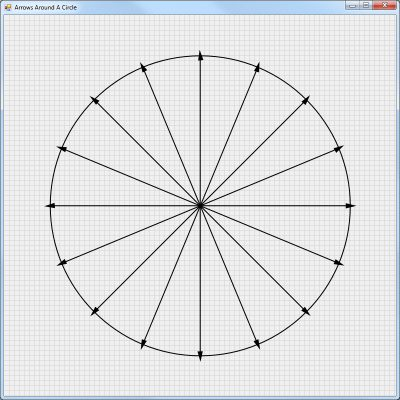# Converting A Processing Sketch To C#

I have invested a lot of time and effort into learning Processing. I mostly use the JavaScript version of Processing since I can then include working demos in my technology notes which are in the form of HTML files compiled into a Microsoft Document Help Collection. I like Processing because it allows me to be creative using my existing programming skills. It has encouraged me to learn more about computer graphics.

Recently I created a Processing sketch to draw direction arrows around a circle. One of my favorite Processing tricks is to divide a circle into equal parts, like pie slices, by finding points around the circle. The purpose of this sketch was to draw direction arrows rotated in the proper direction to point towards these points around the circle. I needed this to illustrate some sketches for directed graphs, a type of graph in Graph Theory. But orienting objects around a circle is a generally useful trick.

My Processing sketch for drawing arrows around a circle can be found at: https://www.openprocessing.org/sketch/1031385

The challenge in recreating this sketch in C# is to find an alternative to the matrix stack as implemented in Processing. Processing provides the handy functions pushMatrix() and popMatrix() as explained at https://processing.org/reference/pushMatrix_.html. C# supports creating a matrix and methods to invert, rotate, scale, and transform matrices. You can then apply the transformation to the graphics object. But there isn’t a matrix stack so it is hard to figure out how to restore the prior coordinate system. After a lot of trial and error I figured out a way to make this work. The trick is to reset the matrix and then apply the transformation back to the graphics object at just the right point in the code.

As I said, this required a lot of trial and error and might not work for a different Processing sketch. You will have to experiment with when to draw things and when to apply transformations. But I was able to recreate my Processing sketch using the C# code shown below. The arrow heads are not perfectly aligned because the `DrawPolygon` method in C# is not an exact replacement for the `beginShape` method in Processing.

``````
using System;
using System.Collections.Generic;
using System.ComponentModel;
using System.Data;
using System.Drawing;
using System.Drawing.Drawing2D;
using System.Globalization;
using System.Linq;
using System.Text;
using System.Windows.Forms;

namespace ArrowsAroundCircle
{
public partial class Form1 : Form
{
// A global variable
System.Drawing.Graphics graphicsObj;

public Form1()
{
InitializeComponent();
}

private void Form1_Paint(object sender, PaintEventArgs e)
{
graphicsObj = this.CreateGraphics();

// Set the SmoothingMode property to smooth the lines.
graphicsObj.SmoothingMode = System.Drawing.Drawing2D.SmoothingMode.AntiAlias;

// Create a grid
int gridSize = 10;

for (int x = gridSize; x <= Form1.ActiveForm.Width; x += gridSize)
{
for (int y = gridSize; y <= Form1.ActiveForm.Height; y += gridSize)
{

// Draw a grid
Pen objPen = new Pen(System.Drawing.Color.LightGray, 1);
graphicsObj.DrawLine(objPen, x, 0, x, Form1.ActiveForm.Height);
graphicsObj.DrawLine(objPen, x - gridSize, y, Form1.ActiveForm.Width, y);
}
}

// This centers what is drawn
graphicsObj.TranslateTransform(graphicsObj.VisibleClipBounds.Width / 2, graphicsObj.VisibleClipBounds.Height / 2, MatrixOrder.Append);

// Create pen.
Pen blackPen = new Pen(Color.Black, 2);

// Radius is the diameter divided by two
int radius = 600 / 2;

// Draw a set number of points around the radius of the circle to divide the circle into equal parts
int NumberOfPoints = 16;
for (int i = 0; i < NumberOfPoints; i++)
{
double angle = i * ((Math.PI * 2) / NumberOfPoints);
graphicsObj.DrawLine(blackPen, 0, 0, Convert.ToInt32(radius * Math.Cos(angle)), Convert.ToInt32(radius * Math.Sin(angle)));
}

// Draw a circle
// Equivalent to ellipse(0, 0, 600, 600);
Rectangle myRectangle = new Rectangle(-300, -300, 600, 600);
graphicsObj.DrawEllipse(blackPen, myRectangle);

// Draw the arrows around the circle last
for (int i = 0; i < NumberOfPoints; i++)
{
double angle = i * ((Math.PI * 2) / NumberOfPoints);
float degrees = 360 / NumberOfPoints;
ArrowHead(Convert.ToInt32(radius * Math.Cos(angle)), Convert.ToInt32(radius * Math.Sin(angle)), (float)(i * degrees) + 90);
}
}

/// <summary>
/// Draw an arrow head at a set position, rotated at a set angle
/// </summary>
/// <param name="x">The x position.</param>
/// <param name="y">The y position.</param>
/// <param name="t">The angle of rotation.</param>
private void ArrowHead(float x, float y, float t)
{
float r = 4.0F;
float theta = t;

// Create a Matrix object
Matrix X = new Matrix();

x = x + graphicsObj.VisibleClipBounds.Width / 2;
y = y + graphicsObj.VisibleClipBounds.Height / 2;
X.Translate(x, y);
X.Rotate(theta);

// Apply transformation
graphicsObj.Transform = X;

PointF point1 = new PointF(0, -r*2);
PointF point2 = new PointF(-r, r*2);
PointF point3 = new PointF(r, r*2);
PointF[] arrowPoints = { point1, point2, point3 };

// Create pen.
Pen blackPen = new Pen(Color.Black, 2);

// Create a brush
SolidBrush solidBrush = new SolidBrush(Color.Black);
graphicsObj.DrawPolygon(blackPen, arrowPoints);
graphicsObj.FillPolygon(solidBrush, arrowPoints);

// Reset the matrix
X.Reset();

// Apply transformation
graphicsObj.Transform = X;
}

/// <summary>
/// Function to convert degrees to radians
/// </summary>
/// <param name="degrees">degree value</param>
/// <returns>equivalent radian value</returns>
public static double radians(double degrees)
{
double radians = (Math.PI / 180) * degrees;
This will draw the following figure to the window: